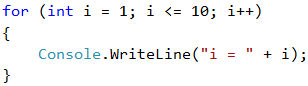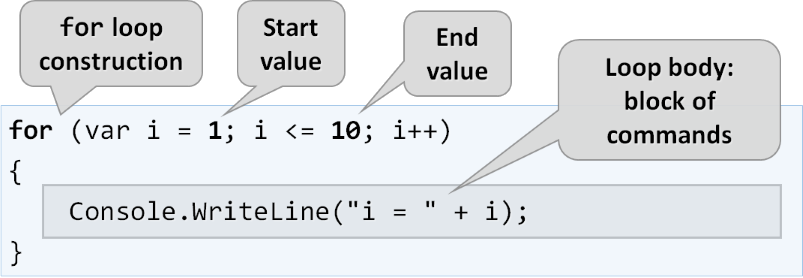# For Loops (Repeating Code Blocks)

In programming it is often required to perform a block of commands multiple times. In order to do that, the so-called loops are used. Let's examine an example of a for loop that passes sequentially through the numbers from 1 to 10 and prints them:The loop starts with the for operator and passes through all values for a particular variable in a given range, for example the numbers from 1 to 10 (included), and for each value it performs a series of commands.

## Video: Simple For-Loops

Watch the video about the for-loop statement: https://youtu.be/yzEamf5L1ZY.

## Syntax: For-Loop

Upon declaring the loop, you can specify a start value and an end value. The body of the loop is usually enclosed in curly brackets { } and represents a block of one or multiple commands. The figure below shows the structure of a for loop:In most cases a for loop is run between 1 and n times (for example from 1 to 10). The purpose of the loop is to pass sequentially through the numbers 1, 2, 3, …, n and for each of them to perform a particular action. In the example above, the i variable accepts values from 1 to 10 and the current value is printed in the body of the loop. The loop repeats 10 times and each of these repetitions is called an "iteration".

## For Loops – Simple Examples

Now, let's demonstrate how to use the for loop in practice by a few simple examples: